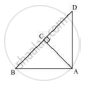Share

# Triangle Abd is a Right Triangle Right-angled at a and Ac ⊥ Bd. Show that - CBSE Class 10 - Mathematics

ConceptApplication of Pythagoras Theorem in Acute Angle and Obtuse Angle

#### Question

∆ABD is a right triangle right-angled at A and AC ⊥ BD. Show that

(i) AB2 = BC x BD

(ii) AC2 = BC x DC

(iii) AD2 = BD x CD

(iv) "AB"^2/"AC"^2="BD"/"DC"

#### Solution∠DAB = ∠ACB = 90°

∠ABD = ∠CBA                       (common angle)

So, ΔADB ~ ΔCAB                (by AAA similarity)

Therefore "AB"/"CB"="BD"/"AB"

⇒ AB2 = CB × BD

(ii) Let ∠CAB = x

In ΔCBA

∠CBA = 180° − 90° − 𝑥

∠CBA = 90° − 𝑥

∠CDA = 90° − ∠CAB

= 90° − 𝑥

∠CDA = 180° −90° − (90° − 𝑥)

∠CDA = x

Now in ΔCBA and ΔCAD we may observe that

∠CAB = ∠CDA

∠ACB = ∠DCA = 90°

Therefore ΔCBA ~ ΔCAD (by AAA rule)

Therefore "AC"/"DC"="BC"/"AC"

⇒ AC2 = DC × BC

(iii) In ΔDCA & ΔDAB

∠DCA = ∠DAB (both are equal to 90°)

∠DAC = ∠DBA (remaining angle)

ΔDCA ~ ΔDAB (AAA property)

Therefore "DC"/"DA"="DA"/"DB"

(iv) From part (i) 𝐴𝐵2 = 𝐶𝐵 × 𝐵𝐷

From part (ii) 𝐴𝐶2 = 𝐷𝐶 × 𝐵𝐶

Hence "AB"^2/"AC"^2=(CBxxBD)/(DCxxBC)

"AB"^2/"AC"^2="BD"/"DC"

Hence proved

Is there an error in this question or solution?

#### Video TutorialsVIEW ALL 

Solution Triangle Abd is a Right Triangle Right-angled at a and Ac ⊥ Bd. Show that Concept: Application of Pythagoras Theorem in Acute Angle and Obtuse Angle.
S